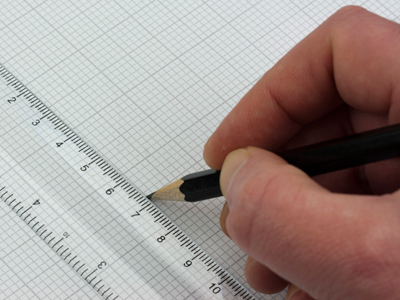# Position 3 (Medium)

You have made it to the third of our Medium level Eleven Plus maths quizzes on Position – give yourself a pat on the back! In the previous two quizzes we introduced you to the x and y axes on a graph, and showed you how to place and to read coordinates. In this quiz we’ll give you more opportunity to practice with coordinates.

You’ll find that some of these questions are very similar to ones you’ve already answered. That is deliberate. The more you practice this topic, the better you will do in your exams. Many students struggle with Position and it is important that you understand the four quadrants on a graph, which of the coordinates to read first, and how to draw shapes with coordinates.

If you haven’t already played the previous quizzes on Position, then please do so before you try this one. Once you’ve mastered all the Medium quizzes, only then should you move on to the Difficult ones. Good luck!

1.
Line A is drawn through the points (6, 0) and (0, 6), and line B is drawn through the points (0, 0) and (6, 6). Which of the following statements about these lines is correct?
The lines intersect at an acute angle
The lines intersect at an obtuse angle
The lines intersect at right angles
The lines are parallel
If you draw the lines, you will see that both lines pass through the point (3, 3) at right angles
2.
The second quadrant of the coordinate plane contains all the points (x, y) where the x value is negative and the y value is positive. Which of the following points lies in the second quadrant?
(8, -6)
(-8, -6)
(-8, 6)
(8, 6)
The x value is negative and the y value is positive
3.
What shape do you get if you join up the points (-1, -1), (-5, -1), (-5, -8) with straight lines and in which quadrant does it lie in?
A triangle in the first quadrant
A triangle in the second quadrant
A triangle in the third quadrant
A triangle in the fourth quadrant
There are three coordinates, all of which are negative, so the shape is a triangle in the third quadrant
4.
What shape do you get if you join up the points (0, 0), (3, 0), (3, -1), (0, -1) with straight lines and in which quadrant does it lie in?
A square in the fourth quadrant
A rectangle in the fourth quadrant
A rectangle in the second quadrant
A rectangle in the first quadrant
(0, 0), (3, 0) and (0, -1) lie on the coordinate axes, but (3, -1) lies in the fourth quadrant and this makes the rectangle lie in this quadrant. It can’t be a square because its sides are not the same length
5.
The first quadrant of the coordinate plane contains all the points (x, y) where both the x and y values are positive. Which of the following points lies in the first quadrant?
(2, 8)
(2, -8)
(-2, 8)
(-2, -8)
The x and y values are BOTH positive
6.
Where does the point (18, 0) lie on the coordinate plane?
It lies on the positive part of the x-axis to the left of the origin (0, 0)
It lies on the positive part of the x-axis to the right of the origin (0, 0)
It lies on the negative part of the x-axis to the right of the origin (0, 0)
It lies on the negative part of the x-axis to the left of the origin (0, 0)
(x, y) = (18, 0): the y value is 0, so the point lies at 18 on the positive part of the x-axis
7.
The third quadrant of the coordinate plane contains all the points (x, y) where both the x value and the y value are negative. Which of the following points lies in the third quadrant?
(11, 14)
(-11, 14)
(11, -14)
(-11, -14)
Both the x value and the y value are negative
8.
A circle with centre (0, 0) and diameter 6 cm is drawn on the coordinate plane. Which points will the circle pass through?
(6, 0), (0, 6), (-6, 0), (0, -6)
(3, 0), (0, 3), (-3, 0), (0, -3)
(6, 0), (6, 6), (-6, 0), (0, -6)
(3, 0), (3, 3), (-3, 0), (0, -3)
The radius is half the diameter = 3 cm; therefore, the circle will pass through those points that are at a distance of 3 cm from its centre (0, 0)
9.
The fourth quadrant of the coordinate plane contains all the points (x, y) where the x value is positive and the y value is negative. Which of the following points lies in the fourth quadrant?
(-10, -4)
(10, 4)
(-10, 4)
(10, -4)
The x value is positive and the y value is negative
10.
Where does the point (0, -7) lie on the coordinate plane?
It lies on the negative part of the y-axis below the origin (0, 0)
It lies on the negative part of the y-axis above the origin (0, 0)
It lies on the positive part of the y-axis above the origin (0, 0)
It lies on the positive part of the y-axis below the origin (0, 0)
(x, y) = (0, -7): the x value is 0, so the point lies at -7 on the negative part of the y-axis
Author:  Frank Evans